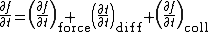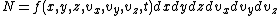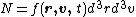# Boltzmann transport equation

## Boltzmann transport equation

An equation which is used to study the nonequilibrium behavior of a collection of particles. In a state of equilibrium a gas of particles has uniform composition and constant temperature and density. If the gas is subjected to a temperature difference or disturbed by externally applied electric, magnetic, or mechanical forces, it will be set in motion and the temperature, density, and composition may become functions of position and time; in other words, the gas moves out of equilibrium. The Boltzmann equation applies to a quantity known as the distribution function, which describes this nonequilibrium state mathematically and specifies how quickly and in what manner the state of the gas changes when the disturbing forces are varied. See Kinetic theory of matter

Equation (1)

(1)is the Boltzmann transport equation shown below, where f is the unknown distribution function which, in its most general form, depends on a position vector r , a velocity vector v , and the time t. The quantity ∂f/∂t on the left side of Eq. (1) is the rate of change of f at fixed values of r and v . The equation expresses this rate of change as the sum of three contributions: first, (∂f/∂t)force arises when the velocities of the particles change with time as a result of external driving forces; second, (∂f/∂t)diff is the effect of the diffusion of the particles from one region in space to the other; and third, (∂f/∂t)coll is the effect of the collisions of the particles with each other or with other kinds of particles.

The distribution function carries information about the positions and velocities of the particles at any time. The probable number of particles N at the time t within the spatial element dxdydz located at (x, y, z) and with velocities in the element dvxdvydvz at the point (vx, vy, vz) is given by Eq. (2) or, in vector notation, by Eq. (3).

(2)(3)It is assumed that the particles are identical; a different distribution function must be used for each species if several kinds of particles are present.

The Boltzmann equation is irreversible in time in the sense that if f( r , v , t) is a solution, then f( r , - v , -t) is not a solution. Thus if an isolated system is initially not in equilibrium, it approaches equilibrium as time advances; the time-reversed performance, in which the system departs farther from equilibrium, does not occur. This is paradoxical because actual physical systems are reversible in time when looked at on an atomic scale. From a mathematical point of view it is puzzling that one can begin with the exact equations of motion, reversible in time, and by making reasonable approximations arrive at the irreversible Boltzmann equation. The resolution of this paradox lies in the statistical nature of the Boltzmann equation. It does not describe the behavior of a single system, but the average behavior of a large number of systems.

The Boltzmann equation can be used to calculate the electronic transport properties of metals and semiconductors. For example, if an electric field is applied to a solid, one must solve the Boltzmann equation for the distribution function of the electrons. If the electric field is constant, the distribution function is also constant and is displaced in velocity space in such a way that fewer electrons are moving in the direction of the field than in the opposite direction. This corresponds to a current flow in the direction of the field. See Free-electron theory of metals

With the Boltzmann equation one can also calculate the heat current flowing in a solid as the result of a temperature difference, the constant of proportionality between the heat current per unit area and the temperature gradient being the thermal conductivity. In still more generality, both an electric field and a temperature gradient can be applied. The resulting equations describe thermoelectric phenomena, such as the Peltier and Seebeck effects. Finally, if a constant magnetic field is also applied, it is found that the electrical conductivity usually decreases with increasing magnetic field, a behavior known as magnetoresistance. These equations also describe the Hall effect, as well as more complex thermomagnetic phenomena, such as the Ettingshausen and Nernst effects.

Nonequilibrium properties of atomic or molecular gases such as viscosity, thermal conduction, and diffusion have been treated with the Boltzmann equation. Although many useful results, such as the independence of the viscosity of a gas on pressure, can be obtained by simple approximate methods, the Boltzmann equation must be used in order to obtain quantitatively correct results. See Diffusion, Viscosity

If one proceeds from a neutral gas to a charged gas or plasma, with the electrons partially removed from the atoms, a number of new phenomena appear. As a consequence of the long-range Coulomb forces between the charges, the plasma can exhibit oscillations in which the free electrons move back and forth with respect to the relatively stationary heavy positive ions at the characteristic frequency known as the plasma frequency. A plasma reflects an electromagnetic wave at a frequency lower than the plasma frequency, but transmits the wave at a higher frequency. This fact explains many characteristics of long-distance radio transmission, made possible by reflection of radio waves by the ionosphere, a low-density plasma. See Plasma (physics)

If a magnetic field is applied to the plasma, its motion can become complex. In an Alfvén wave, which propagates in the direction of the magnetic field, the magnetic field lines oscillate like stretched strings, while waves that propagate in a direction perpendicular to the magnetic field have quite different properties. The outstanding problem in the attainment of a controlled thermonuclear reaction is to design a magnetic field configuration that can contain an extremely hot plasma long enough to allow nuclear reactions to take place. Plasmas in association with magnetic fields also occur in many astronomical phenomena. See Magnetohydrodynamics

Many properties of plasmas can be calculated by studying the motion of individual particles in electric and magnetic fields, or by using hydrodynamic equations or the Vlasov equation, together with Maxwell's equations. However, subtle properties of plasmas, such as diffusion processes and the damping of waves, can best be understood by starting with the Boltzmann equation or the closely related Fokker-Planck equation. See Maxwell's equations

McGraw-Hill Concise Encyclopedia of Physics. © 2002 by The McGraw-Hill Companies, Inc.

## Boltzmann transport equation

[′bōlts·mən ′tranz‚pȯrt i′kwā·zhən]
(statistical mechanics)
An equation used to study the nonequilibrium behavior of a collection of particles; it states that the rate of change of a function which specifies the probability of finding a particle in a unit volume of phase space is equal to the sum of terms arising from external forces, diffusion of particles, and collisions of the particles. Also known as Maxwell-Boltzmann equation.
McGraw-Hill Dictionary of Scientific & Technical Terms, 6E, Copyright © 2003 by The McGraw-Hill Companies, Inc.
References in periodicals archive ?
The Boltzmann transport equation  is central to the neutral particle transport problems as encountered in the field of nuclear engineering community.
Bonini, "Thermoelectric coefficients of n -doped silicon from first principles via the solution of the Boltzmann transport equation," Physical Review B--Condensed Matter and Materials Physics, vol.
The biological tissue is modeled by using the diffusion equation that is Boltzmann transport equation (BTE).
Bypassing the density of states technique used in previous studies of magneto thermoelectric power, Ghatak offers a more fundamental account, because the Boltzmann transport equation, which controls the study of the charge transport properties of the semiconductor devices, can be solved if and only if the E-k dispersion relation is known, which the density of states approach cannot provide.
Because magnons behave much like electrons in this aspect, the researchers developed a theory of magnon transport based on a widely established equation for electron transport in thermoelectrics, called the Boltzmann transport equation. From their derivations, Liao, Zhou, and Chen came up with two new equations to describe magnon transport.
Such transport could in principle be described by means of Boltzmann transport equation (BTE) for charge carriers.
Wang, "Dual-phase-lagging heat conduction based on Boltzmann transport equation," International Journal of Heat and Mass Transfer, vol.
His topics include the growth of semiconductor thin films, potential barrier with a finite height, density of states, the optical absorption coefficient of the interband transition in quantum wells, the Boltzmann transport equation, hetero-junction field effect transistors, and light-emitting diodes.
For accurate investigation of quantum transport in semiconductors, the stochastic solution of the Boltzmann transport equation using the particle Monte Carlo method has become very popular.
DRAGON 4 is a lattice code developed to solve Boltzmann transport equation in two and three dimensions, to apply self-shielding effects and to compute few-group macroscopic cross sections and diffusion coefficients.
Analytic methods incorporate the Boltzmann transport equation (BTE), Green's theorem, and so on.

Site: Follow: Share:
Open / Close